書名: Mathematics Today, A Programmed Course 2A (First Edition), Exercise 1A 作者: K. S. Leung, M. K. Lai 出版社: Canotta Publishing Co. Ltd. 目錄: Home>Math...>A>

 編號 問題 1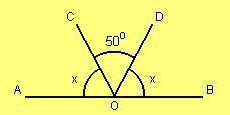Find x. 2Find y. 3Find x, y, z. 4Prove that AB // CD in the figure. 5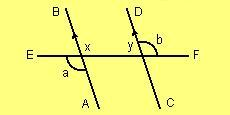In the figure, a = b. Prove that AB // CD. 6In the figure, AB and CD meet at O. EO ^ AB and Ð EOD = 55o. Find b and c. 7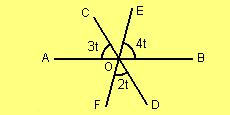In the figure, the three straight lines AB, CD and EF meet at a point O.Find t. 8In the figure, Ð AOC = Ð BOD = 90o and ÐCOD = 38o.Find a, b and c. 9In the figure, AB // CD // EF.The transversal PT cuts AB, CD and EF at Q, R and S respectively.Given that ÐAQR = 40o, find x and y. 10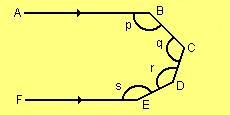In the figure, AB // FE. Find p + q + r + s. 11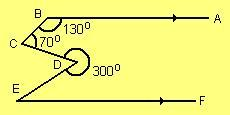In the figure, BA // EF, Ð ABC = 130o, ÐBCD = 70o and reflex ÐCDE = 300o.Find ÐDEF. 12Given the figure as shown.    (a) Find the measure of m.    (b) Prove that AB // XY. 13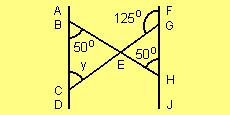Refer to the given figure.    (a) Prove that AD // FJ.    (b) Find the measure of y. 14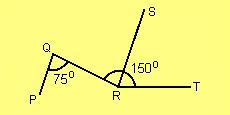Refer to the given figure.Given that ÐPQR = 75o, ÐQRT = 150o and RS is the angle bisector of ÐQRT.Prove that PQ // RS. 15Refer to the figure. Given that p = q and AD // BC.Prove that BA // CD. 16Refer to the figure. Prove that    (a) AB // CD    (b) BC // DF 17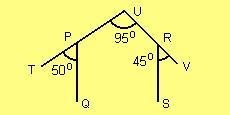In the given figure, ÐTPQ = 50o, ÐPUR = 95o and ÐSRV = 45o.Prove that PQ // RS. 18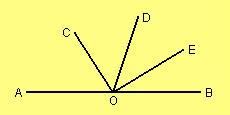In the figure, AOB is a straight line.OC is the angle bisector of ÐAOD and OE is the angle bisector of ÐBOD.Prove that ÐCOE = 90o.